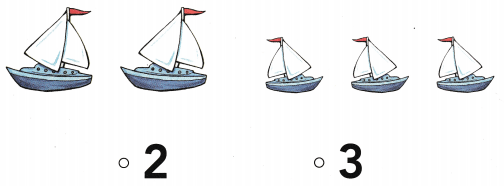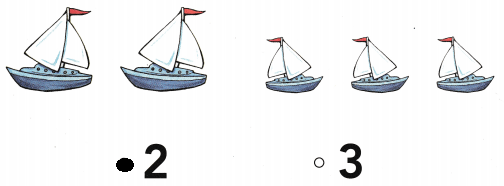# Texas Go Math Kindergarten Lesson 12.3 Answer Key Differences Within 5

Refer to our Texas Go Math Kindergarten Answer Key Pdf to score good marks in the exams. Test yourself by practicing the problems from Texas Go Math Kindergarten Lesson 12.3 Answer Key Differences Within 5.

## Texas Go Math Kindergarten Lesson 12.3 Answer Key Differences Within 5

Explore

DIRECTIONS: There are four birds. One bird flies away. How many birds are left? Trace the circle and X to show the bird flying away from the set. Trace the subtraction sentence.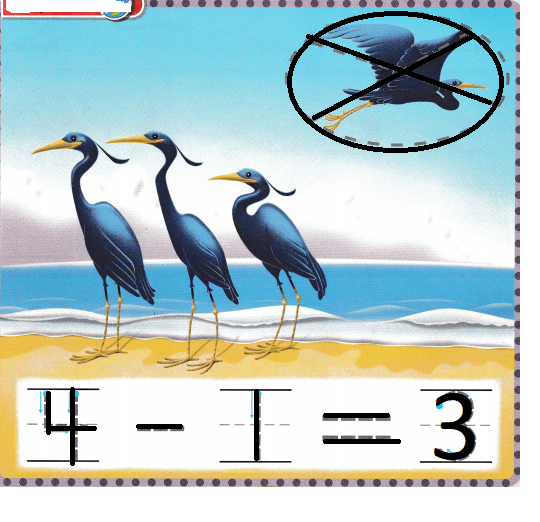Explanation:
There are four birds.
One bird flies away.
4 – 1 = 3
so, 3 birds are still on the shore.

Share and Show

DIRECTIONS: 1. Listen to the subtraction word problem. How many penguins leave? Trace the circle and X. How many penguins are there now? Trace the subtraction sentence. 2-3. Listen to the subtraction word problem. Trace the circle and X. Trace and write to complete the subtraction sentence.

Question 1.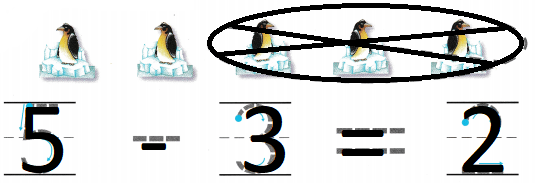Explanation:
There are 5 penguins
3 swim away
so, 2 penguins are there

Question 2.Explanation:
There are 5 ducks
1 duck swim away
so, 4 duck are remaining

Question 3.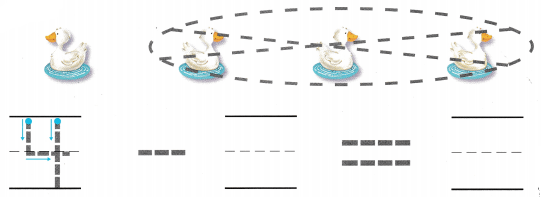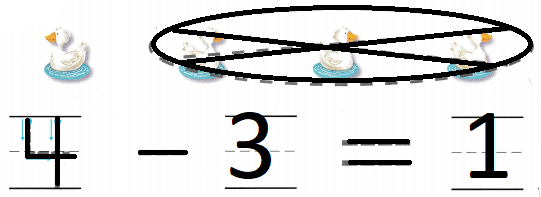Explanation:
There are 4 ducks
3 ducks run away
4 – 3 = 1
so, 1 duck is remaining

DIRECTIONS: 4-6. Listen to the subtraction word problem. Draw a circle and mark an X to show how many leave. Trace and write the numbers to complete the subtraction sentence.

Question 4.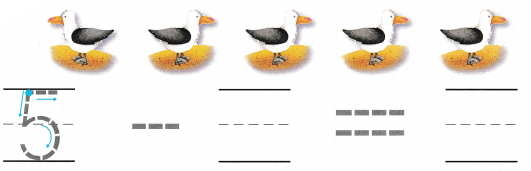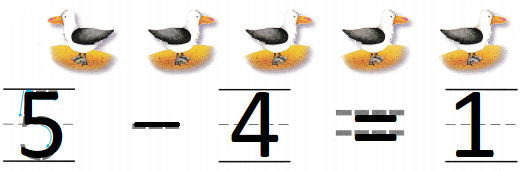Explanation:
There are 5 birds
4 birds leave
5 – 4 = 1
so, 1 bird is still there

Question 5.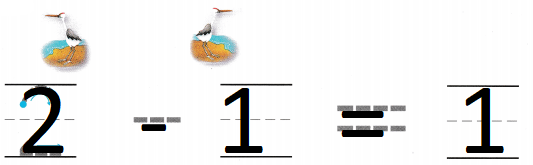Explanation:
There are 2 cranes
1 crane has flied
so, 1 crane is remaining

Question 6.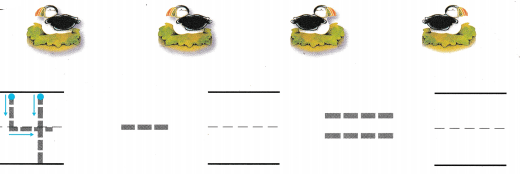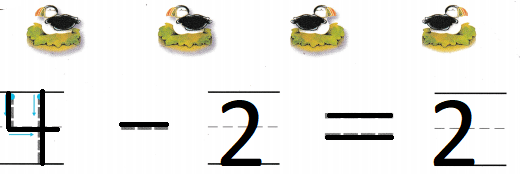Explanation:
There are 4 ducks
2 ducks walked away
4 – 2 = 2
so, 2 ducks are remaining.

HOME ACTIVITY • Have your child tell a word problem for one of the subtraction sentences on this page.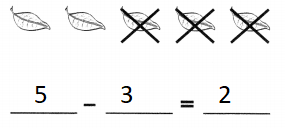Explanation:
There are 5 leaves
3 leaves flew away because of wind
5 – 3 = 2
so, there are 2 more leaves.

DIRECTIONS: 7. Draw to show what you know about this subtraction sentence. Tell a friend about your drawing. Complete the subtraction sentence. 8. Choose the correct answer. There are five birds. Then two of the birds walk away. What is 5 – 2?

Problem Solving

Question 7.Explanation:
There are 3 bananas 2 were eaten
3 – 2 = 1
so, 1 banana is remaining.

Question 8.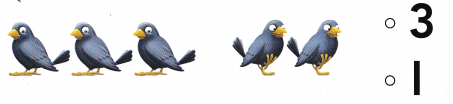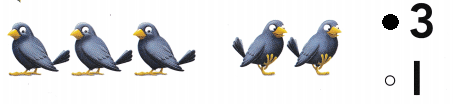Explanation:
There are 5 birds
2 flew away
5 – 2 = 3
so, 3 birds are on the tree.

### Texas Go Math Kindergarten Lesson 12.3 Homework and Practice Answer Key

DIRECTIONS: Listen to the subtraction word problem. Trace the circle and X. Trace and write the numbers to complete the subtraction sentence. 1. There are five sheep. Two of the sheep run away. 2. There are three squirrels. One squirrel runs away.

Question 1.Explanation:
There are five sheep.
Two of the sheep run away
5 – 2 = 3
so, 3 sheep are remaining

Question 2.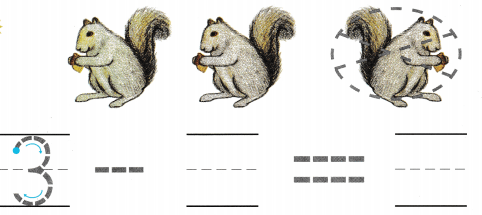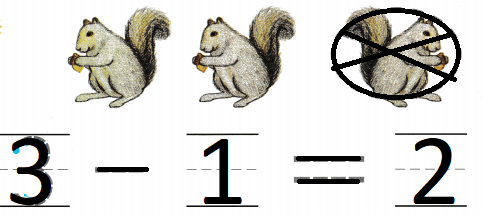Explanation:
There are three squirrels.
One squirrel runs away.
3 – 1 = 2
so, there are 2 squirrels now.

DIRECTIONS: Choose the correct answer. 3. There are four trucks. Three trucks are driven away. What is 4 – 3? 4. There are 5 boats. Three boats sail away. What is 5 – 3?

Lesson Check

Question 3.Explanation:
There are four trucks.
Three trucks are driven away.
4 – 3 = 1
so, 1 truck is left.

Question 4.